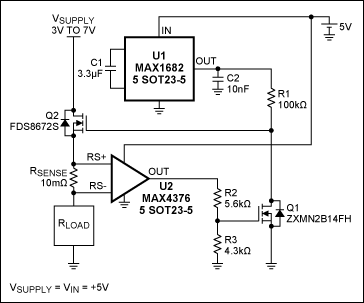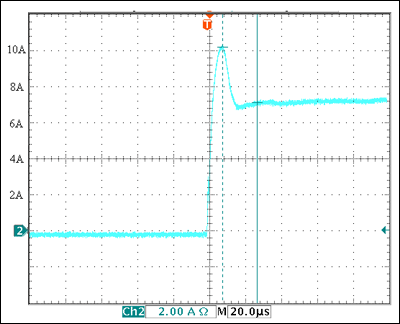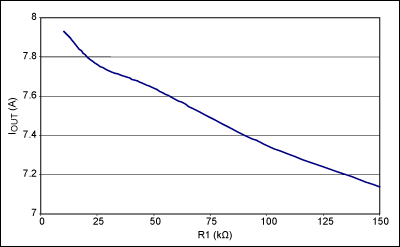# Simple Current Limiter Is Programmable

### 要約

This circuit lets you program a current limit without using microcontrollers or data converters. It consists of a charge-pump voltage doubler (U1), a current-sense amplifier (U2), and two n-channel MOSFETs. The sense-resistor value determines the maximum current limit.

A similar version of this article appeared in the May 18, 2009 issue of EE Times magazine.

Available integrated circuits can let you program a current limit, but they generally involve microcontrollers or data converters (or both). As an alternative, the Figure 1 circuit lets you program a current limit without the intervention of microcontrollers or data converters. It consists of two miniature SOT-5 devices and a few external components. The sense-resistor value determines the maximum current limit.The circuit consists of a charge-pump voltage doubler (U1, MAX1682), a current-sense amplifier (U2, MAX4376), and two n-channel MOSFETs. U1 doubles the supply voltage to provide gate drive for Q2, and U2 amplifies the voltage across the sense resistor (RSENSE) with a gain of 20. This U2 output drives the Q1 gate via the R2/R3 divider, which modulates the current through R1, which in turn sets the Q1 drain voltage and Q2's gate-drive voltage.

U2's output voltage is 20 times the voltage between RS+ and RS-, but has a full-scale limit of 2V. Figure 2 shows the response of this circuit when you suddenly impose a heavy (low resistance) load. It allows an initial current surge to 10A, and then (after 20µs) settles to the desired limit of 7.25A.The maximum allowed in-rush current is set by the sense resistor. A 10mΩ value, for instance, allows a maximum in-rush current of 100mV/10mΩ = 10A. This steady-state limit is determined by the operating characteristics of Q1 and Q2, together with the values chosen for resistors R1–R3. Figure 3 shows the ILIMIT values obtained by varying R1 alone. To set other values of ILIMIT, you can vary the ratio R2/R3 while holding the R1 value fixed.